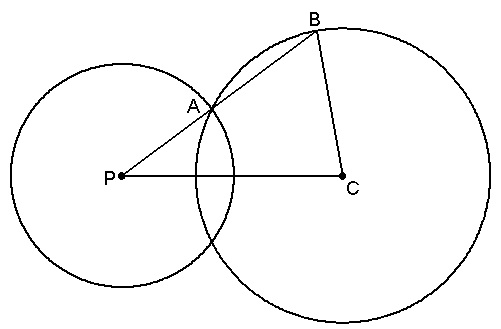# small and big circlesIn the figure shown above, the small circle has its center at $P$ while the big circle has its center at $C$. If $PA=3,BC=4,$ and $PC=6$, what is the length of $AB?$

If your answer is of the form $\frac{a}{b},$ where $a$ and $b$ are coprime positive integers, find $a+b.$

×